# NCERT Solutions for Class 7 Maths Chapter 7 – Congruence of Triangles

Class 7 Maths NCERT Solutions Chapter 7 – Congruence of Triangles comprises of the 2 Exercises

This Chapter contains the Exercises relating to the following topics , which are discussed in Chapter 7 – Congruence of Triangles Class 7 NCERT book  : –

• 7.1 INTRODUCTION
• 7.2 CONGRUENCE OF PLANE FIGURES
• 7.3 CONGRUENCE AMONG LINE SEGMENTS
• 7.4 CONGRUENCE OF ANGLES
• 7.5 CONGRUENCE OF TRIANGLES
• 7.6 CRITERIA FOR CONGRUENCE OF TRIANGLES
• 7.7 CONGRUENCE AMONG RIGHT-ANGLED TRIANGLES

### NCERT Solutions for Class 7 Maths Chapter 7 – Exercise 7.1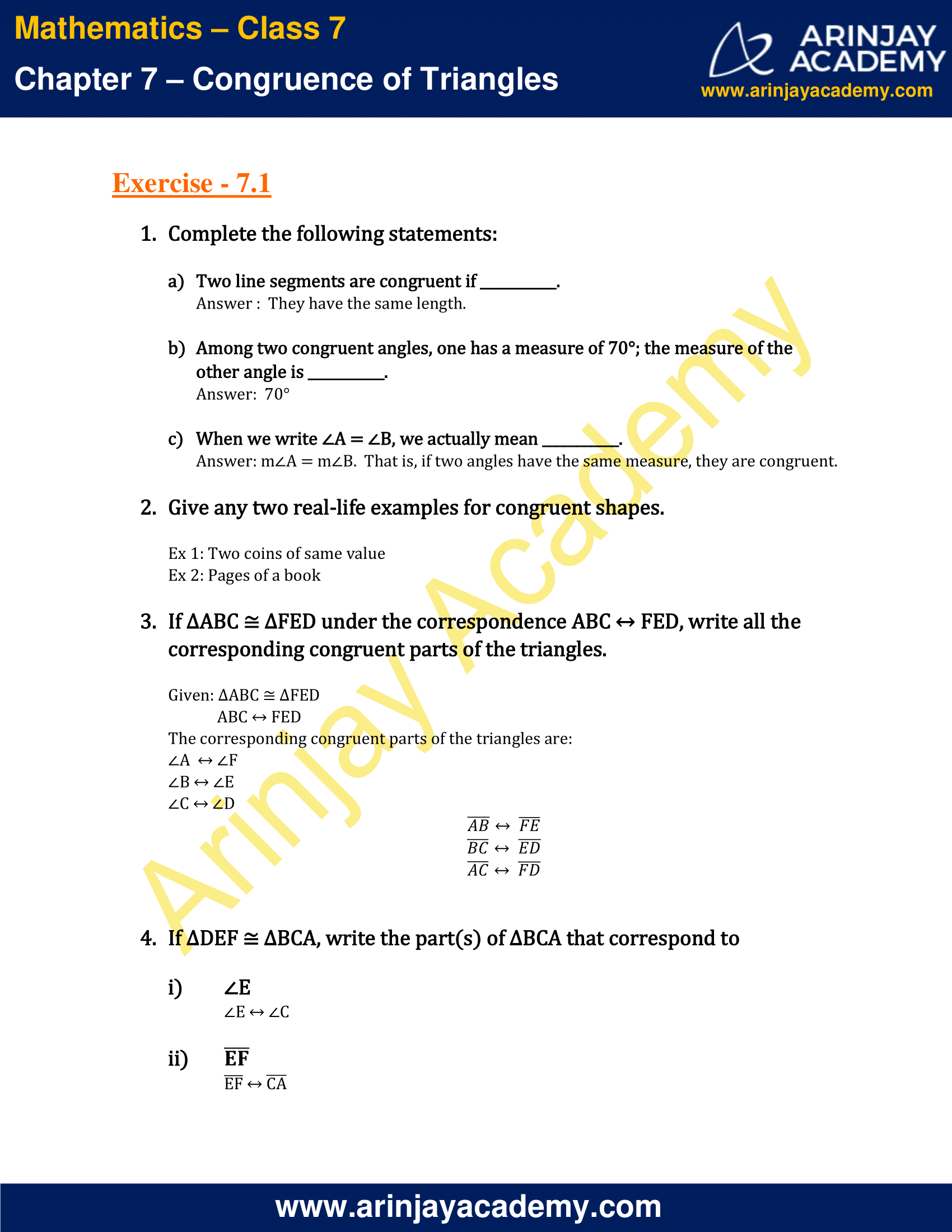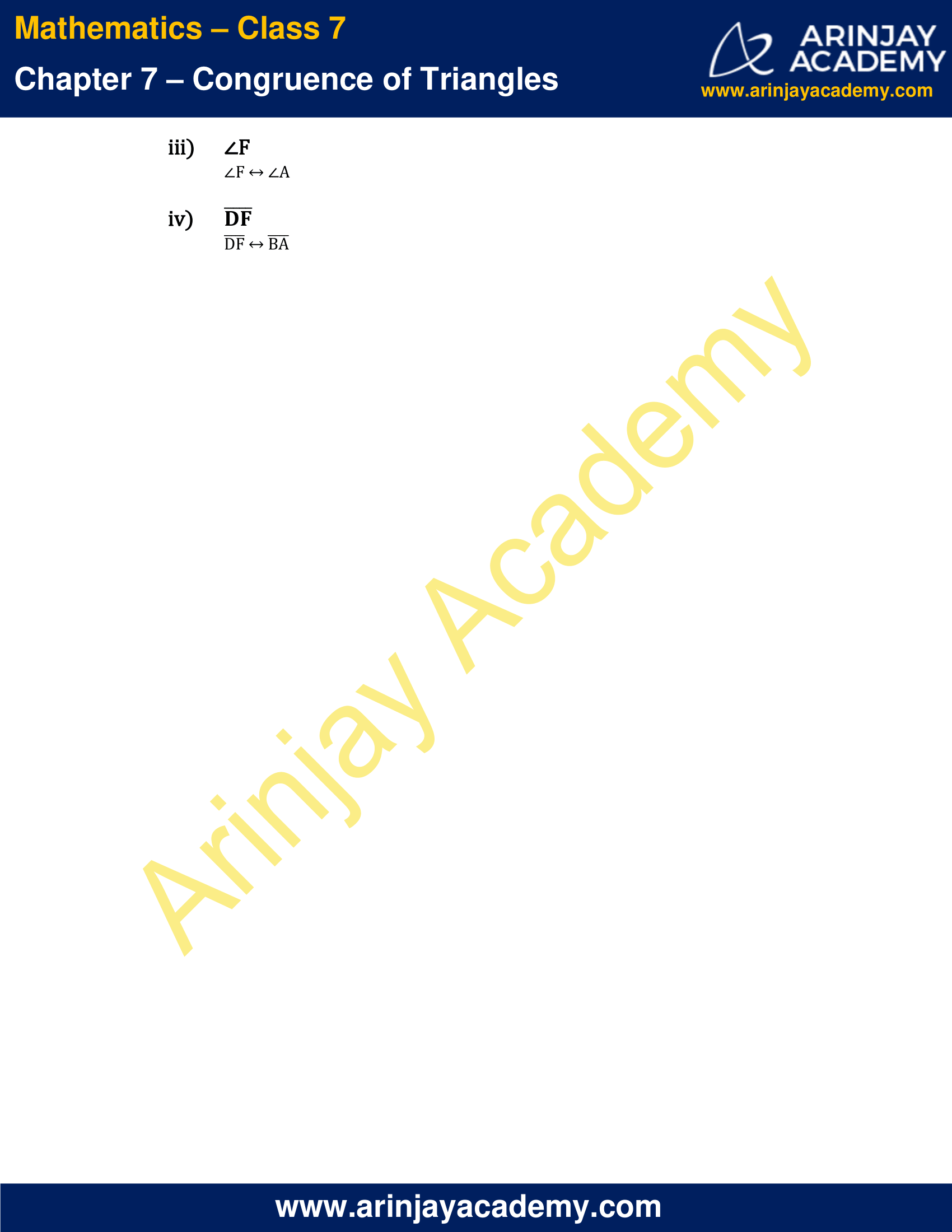### NCERT Solutions for Class 7 Maths Chapter 7 – Exercise 7.2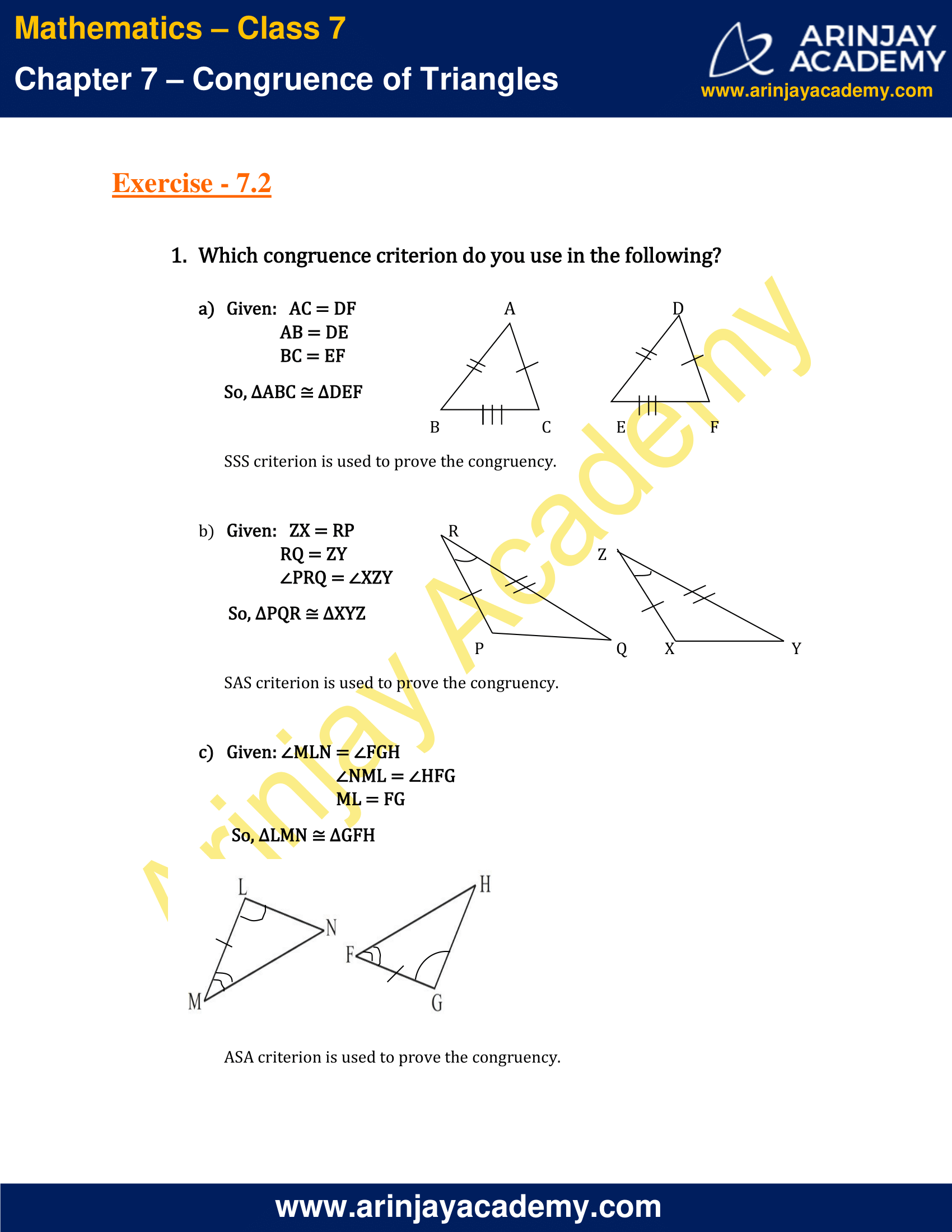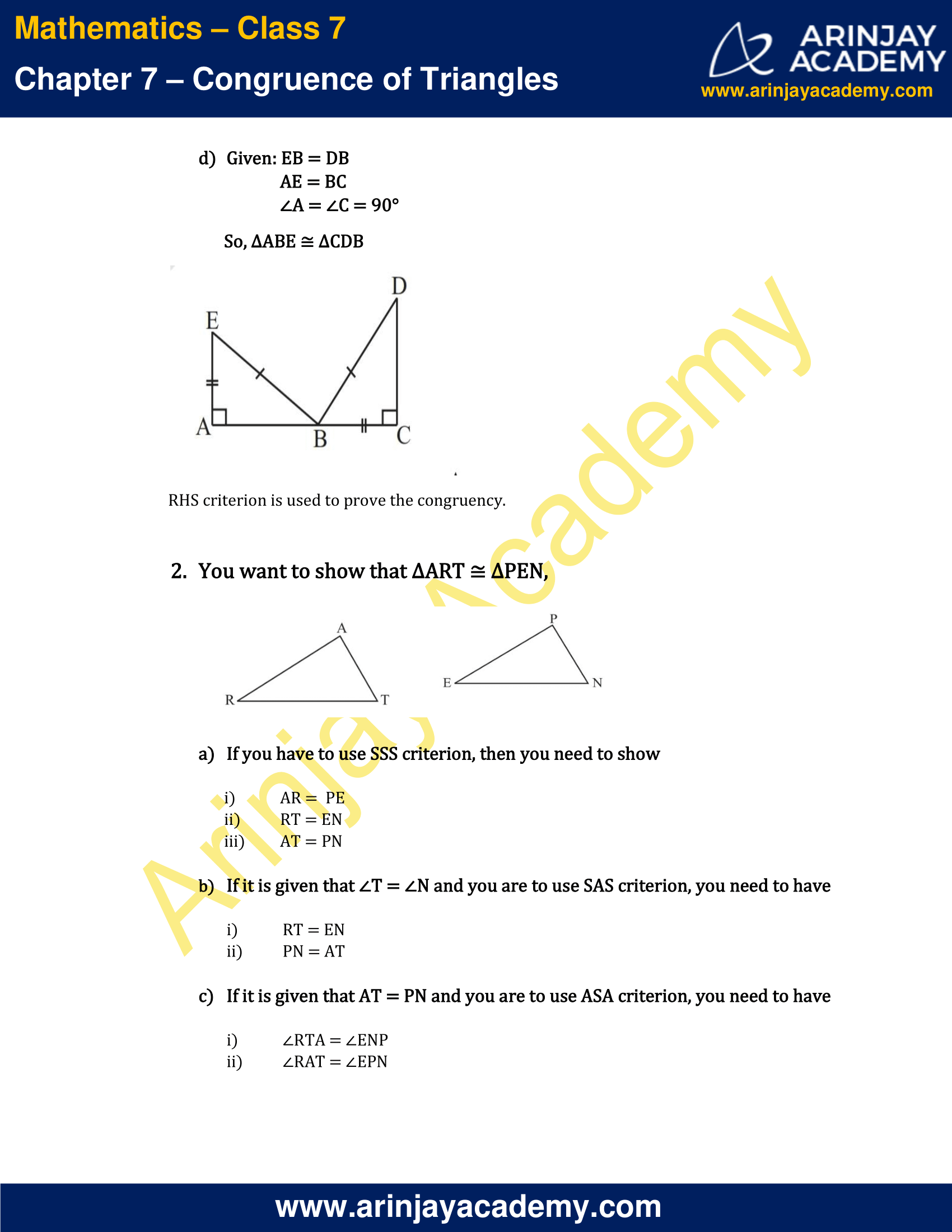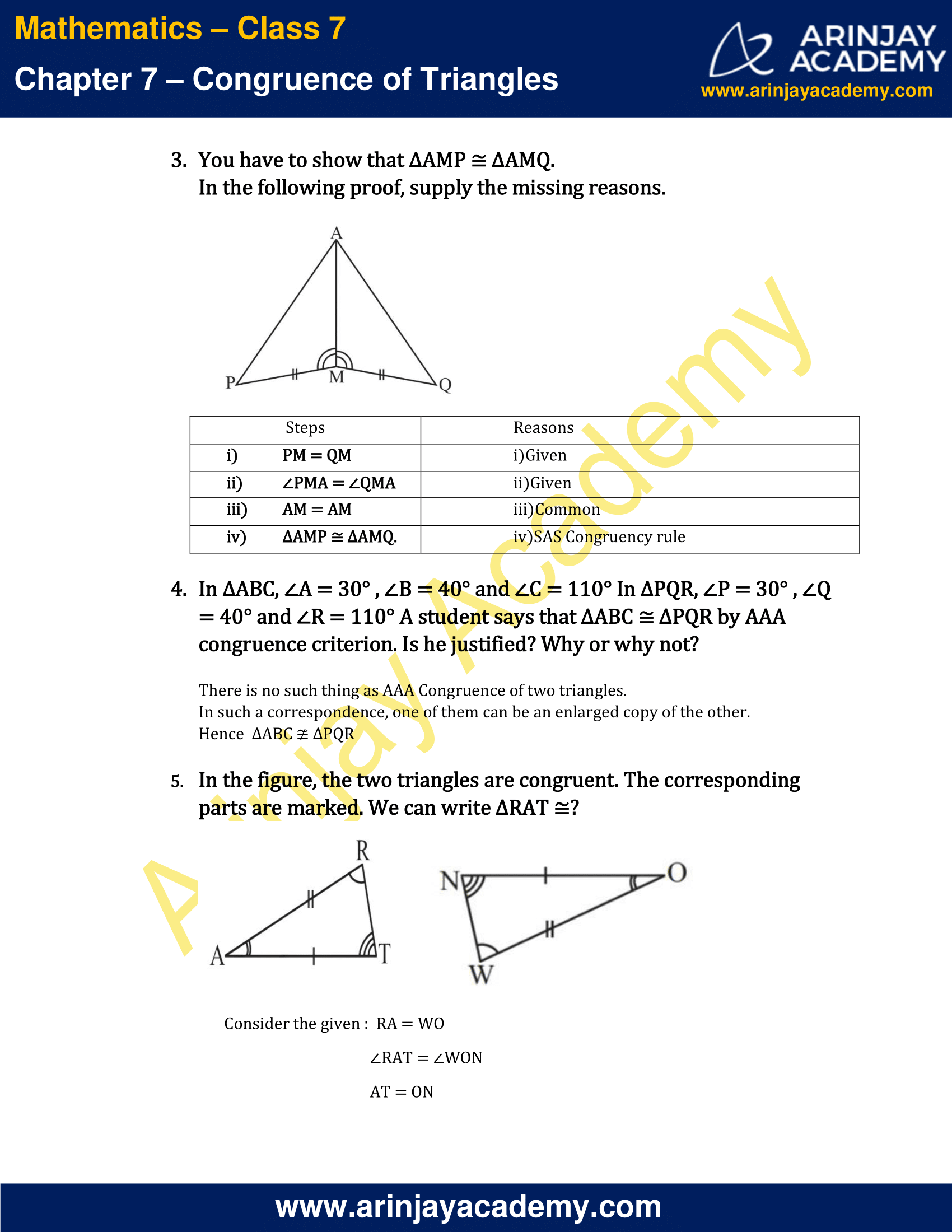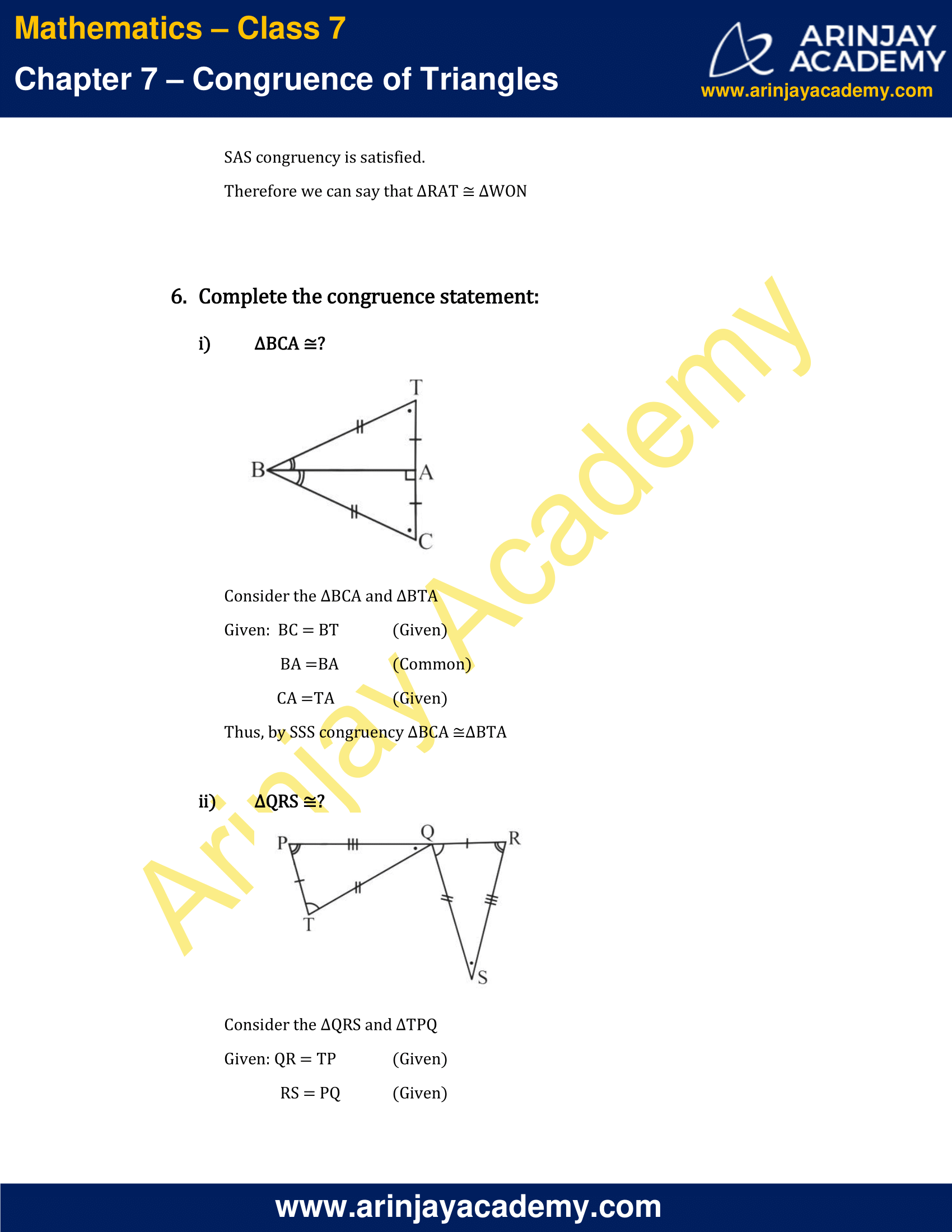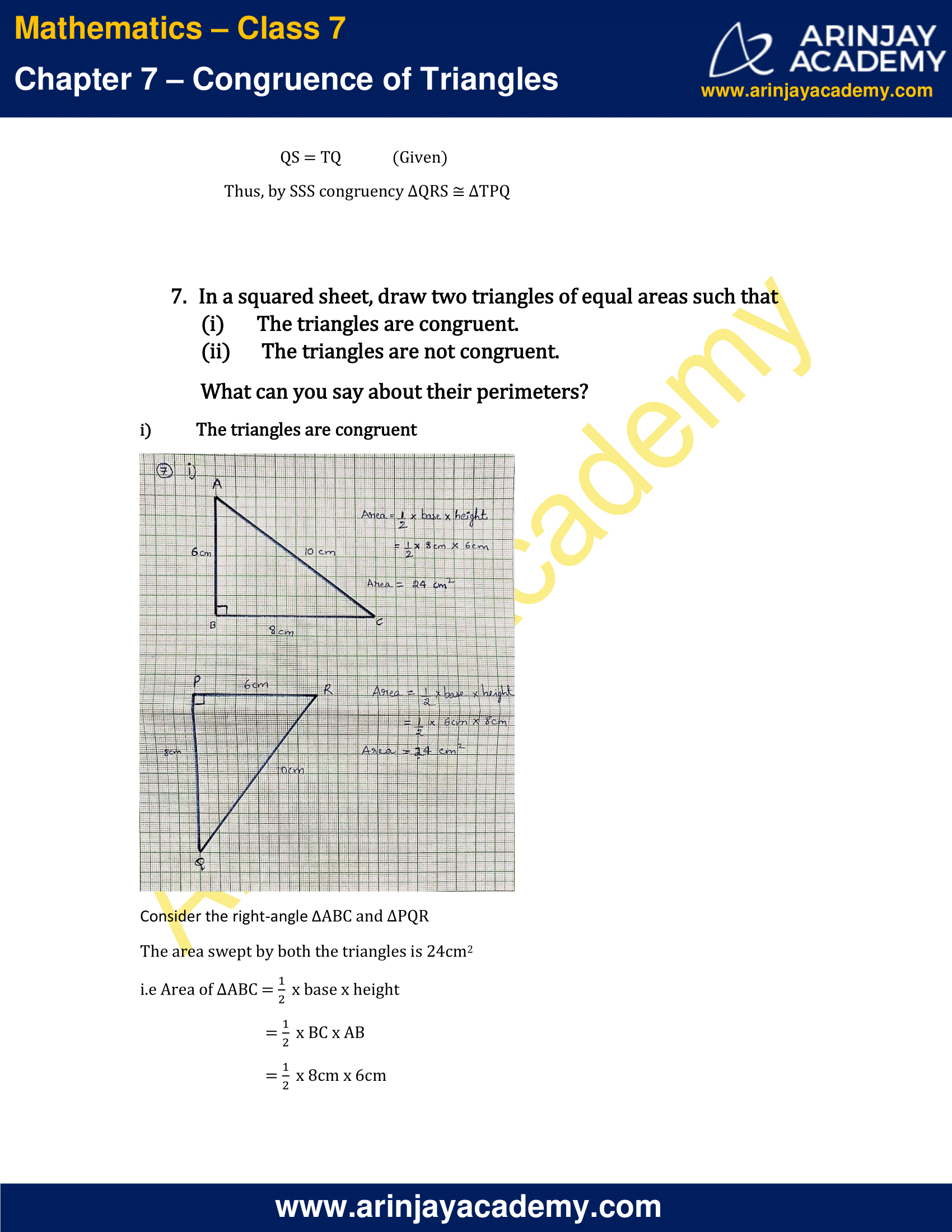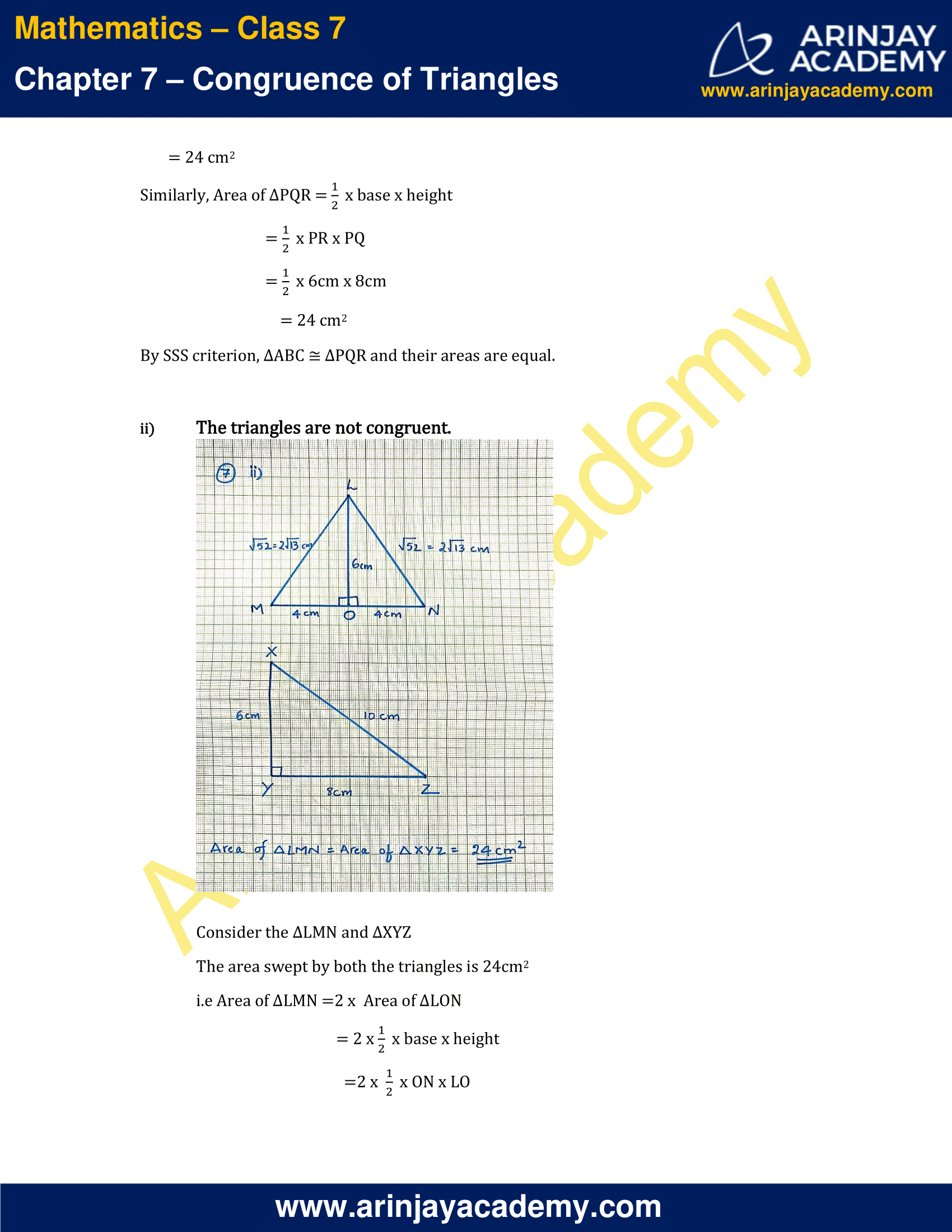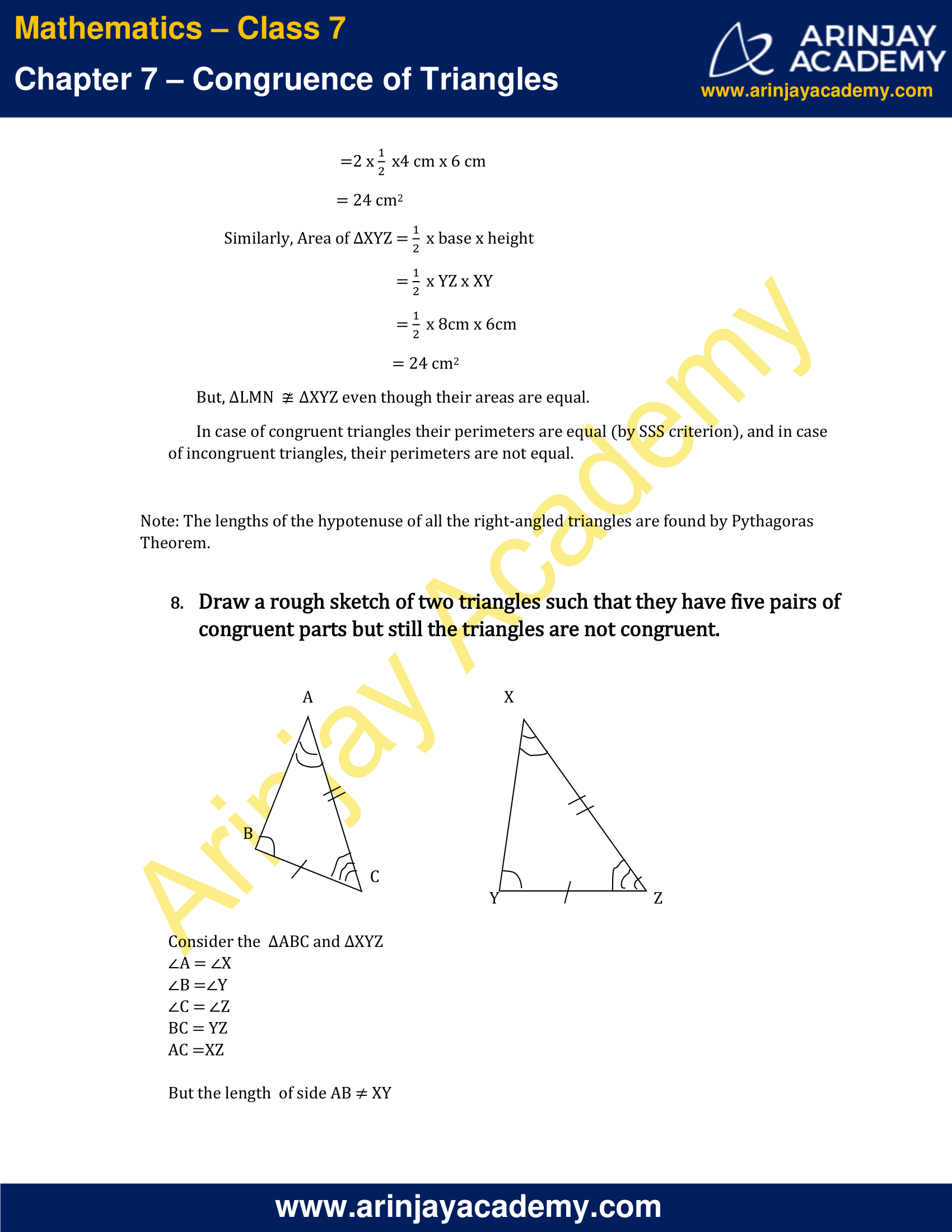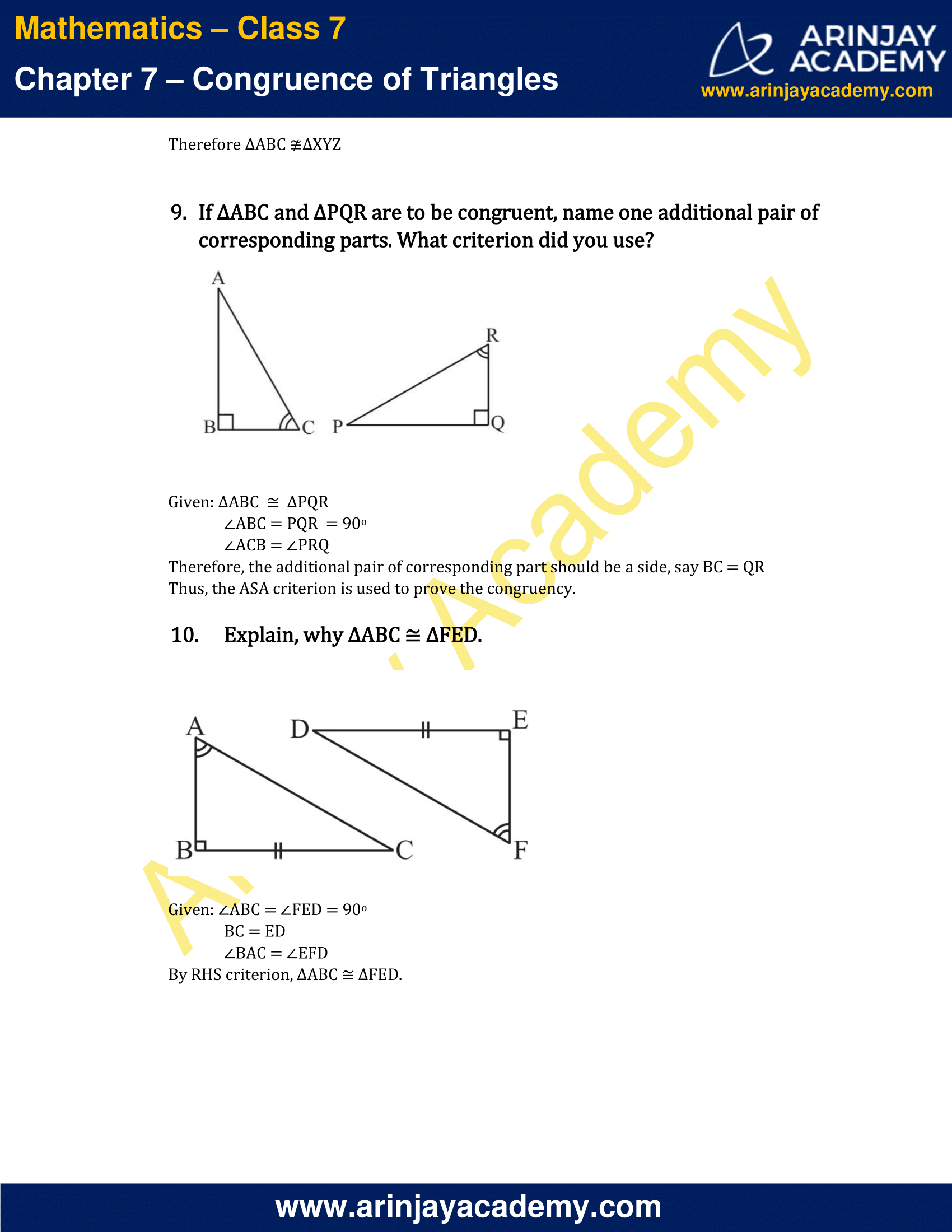### NCERT Solutions for Class 7 Maths Chapter 7 – Exercise 7.1

1. Complete the following statements:

a) Two line segments are congruent if ___________.
Answer :  They have the same length.

b) Among two congruent angles, one has a measure of 70°; the measure of the other angle is ___________.

c) When we write A = B, we actually mean ___________.
Answer: m∠ A = m∠B.  That is, if two angles have the same measure, they are congruent.

2. Give any two real-life examples for congruent shapes.

Ex 1: Two coins of same value
Ex 2: Pages of a book

3. If ∆ABC ∆FED under the correspondence ABC ↔ FED, write all the corresponding congruent parts of the triangles.

Given: ∆ABC ≅ ∆FED
ABC ↔ FED
The corresponding congruent parts of the triangles are:
∠A  ↔ ∠F
∠B ↔ ∠E
∠C ↔ ∠D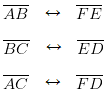4. If ∆DEF ∆BCA, write the part(s) of ∆BCA that correspond to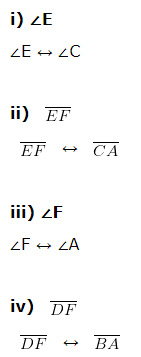### NCERT Solutions for Class 7 Maths Chapter 7 – Exercise 7.2

1. Which congruence criterion do you use in the following?

a) Given: AC = DF
AB = DE
BC = EF
So, ∆ABC ∆DEF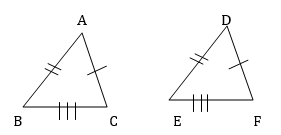SSS criterion is used to prove the congruency.

b) Given: ZX = RP
RQ = ZY
PRQ = XZY
So, ∆PQR
∆XYZ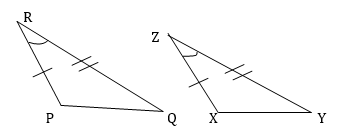SAS criterion is used to prove the congruency.

c) Given: MLN = FGH
NML = HFG
ML = FG
So, ∆LMN ∆GFH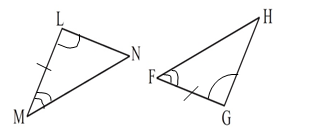ASA criterion is used to prove the congruency.

d) Given: EB = DB
AE = BC
A = C = 90°
So, ∆ABE ∆CDB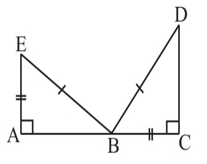RHS criterion is used to prove the congruency.

2. You want to show that ∆ART ∆PEN,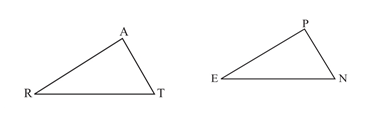a) If you have to use SSS criterion, then you need to show

(i) AR = PE
(ii) RT = EN
(iii) AT = PN

b) If it is given that T = N and you are to use SAS criterion, you need to have

(i) RT = EN
(ii) PN = AT

c) If it is given that AT = PN and you are to use ASA criterion, you need to have

(i) ∠RTA = ∠ENP
(ii) ∠RAT = ∠EPN

3. You have to show that ∆AMP ∆AMQ.
In the following proof, supply the missing reasons.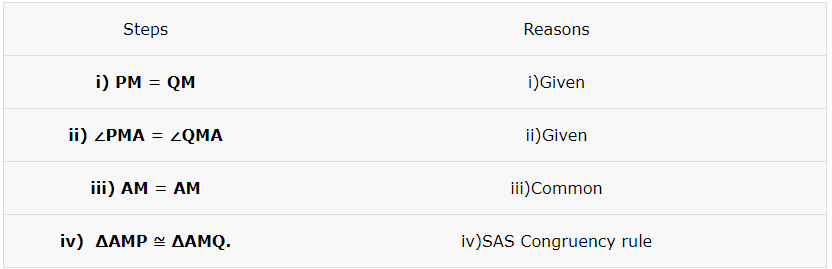4. In ∆ABC, A = 30° , B = 40° and C = 110° In ∆PQR, P = 30° , Q = 40° and R = 110° A student says that ∆ABC ∆PQR by AAA congruence criterion. Is he justified? Why or why not?

There is no such thing as AAA Congruence of two triangles.
In such a correspondence, one of them can be an enlarged copy of the other.
Hence  ∆ABC  ∆PQR

5. In the figure, the two triangles are congruent. The corresponding parts are marked. We can write ∆RAT ?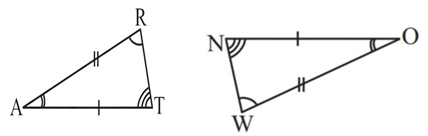Consider the given :  RA = WO
∠RAT = ∠WON
AT = ON
SAS congruency is satisfied.
Therefore we can say that ∆RAT ≅ ∆WON

6. Complete the congruence statement:

i) ∆BCA ?

Consider the ∆BCA and ∆BTA
Given:  BC = BT  (Given)
BA =BA   (Common)
CA =TA   (Given)
Thus, by SSS congruency ∆BCA ≅∆BTA

ii) ∆QRS ?

Consider the ∆QRS and ∆TPQ
Given: QR = TP  (Given)
RS = PQ   (Given)
QS = TQ   (Given)
Thus, by SSS congruency ∆QRS ≅ ∆TPQ

7.  In a squared sheet, draw two triangles of equal areas such that

(i) The triangles are congruent.
(ii) The triangles are not congruent.

What can you say about their perimeters?

Solution:

i) The triangles are congruent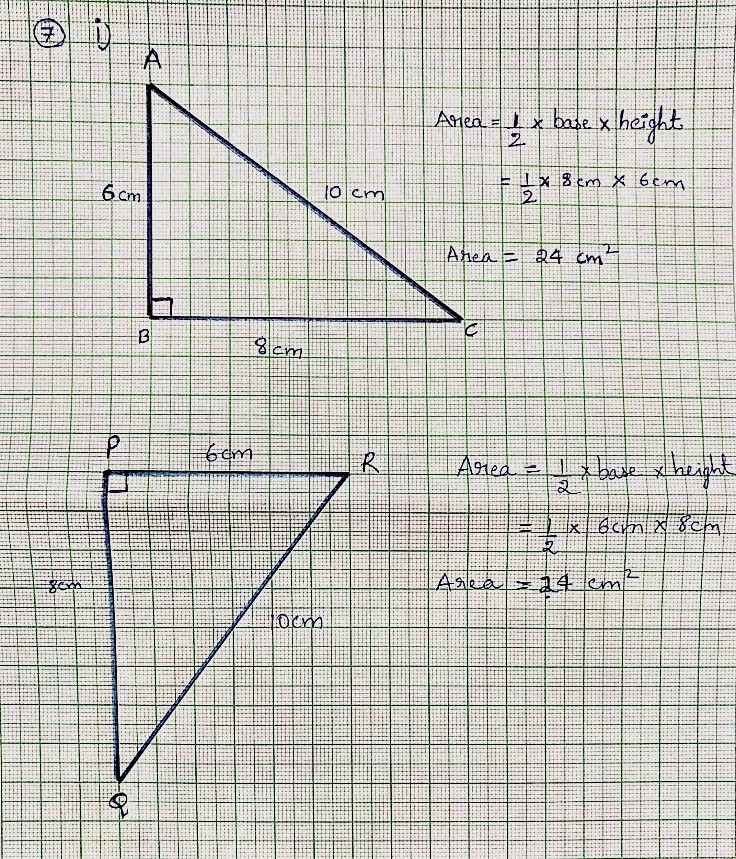Consider the right-angle ∆ABC and ∆PQR

The area swept by both the triangles is 24cm2

i.e Area of ∆ABC = 1/2 x base x height

= 1/2 x BC x AB

= 1/2 x 8cm x 6cm

= 24 cm2

Similarly, Area of ∆PQR = 1/2 x base x height

= 1/2 x PR x PQ

= 1/2 x 6cm x 8cm

= 24 cm2

By SSS criterion, ∆ABC ≅ ∆PQR and their areas are equal.

ii) The triangles are not congruent.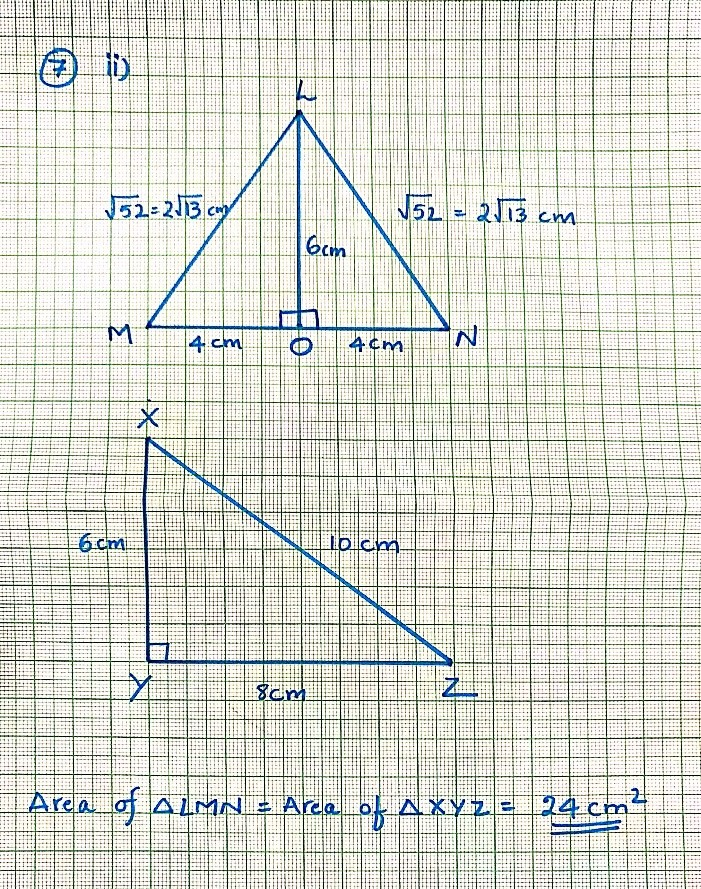Consider the ∆LMN and ∆XYZ

The area swept by both the triangles is 24cm2

i.e Area of ∆LMN =2 x  Area of ∆LON

= 2 x 1/2 x base x height

= 2 x 1/2 x ON x LO

= 2 x 1/2 x 4cm x 6cm

= 24 cm2

Similarly, Area of ∆XYZ = 1/2 x base x height

= 1/2 x YZ x XY

= 1/2 x 8cm x 6cm

= 24 cm2

But, ∆LMN ≇ ∆XYZ even though their areas are equal.

In case of congruent triangles their perimeters are equal (by SSS criterion), and in case of incongruent triangles, their perimeters are not equal.

Note: The lengths of the hypotenuse of all the right-angled triangles are found by Pythagoras Theorem.

8. Draw a rough sketch of two triangles such that they have five pairs of congruent parts but still the triangles are not congruent.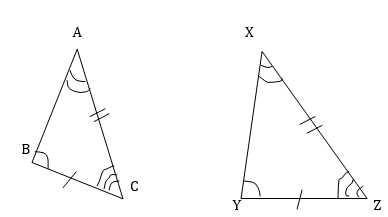Consider the  ∆ABC and  ∆XYZ

∠A = ∠X
∠B =∠Y
∠C = ∠Z
BC = YZ
AC =XZ

But the length  of side AB ≠ XY
Therefore ∆ABC ≇ ∆XYZ

9. If ∆ABC and ∆PQR are to be congruent, name one additional pair of corresponding parts. What criterion did you use?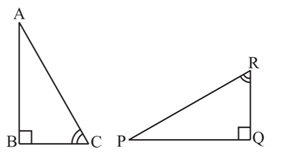Given: ∆ABC  ≅  ∆PQR
∠ABC = PQR  = 90o
∠ACB = ∠PRQ
Therefore, the additional pair of corresponding part should be a side, say BC = QR
Thus, the ASA criterion is used to prove the congruency.

10. Explain, why ∆ABC ∆FED.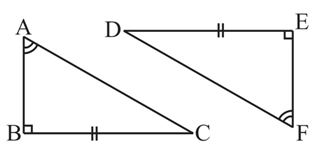Given: ∠ABC = ∠FED = 90o
BC = ED
∠BAC = ∠EFD
By RHS criterion, ∆ABC ≅ ∆FED.

With this we come to the end of NCERT Solutions for Class 7 Maths Chapter 7 Congruence of Triangles. We hope these helped you study your subject.

Download NCERT Solutions for Class 7 Maths Chapter 7 – Congruence of Triangles